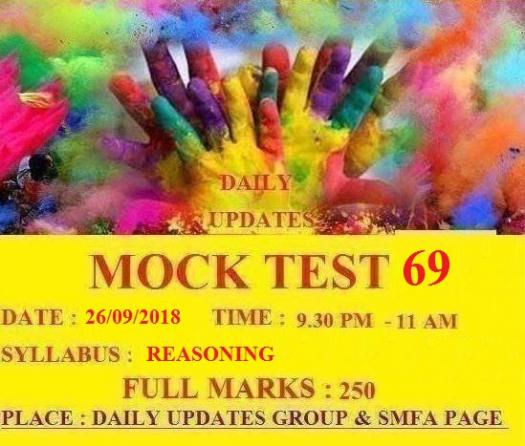# Daily Updates Mock Test 69

50 Questions | Total Attempts: 80Settings• 1.
• A.

12

• B.

13

• C.

14

• D.

15

• 2.
3A 2B 4C 27G ? 64I 9U 4V 16W
• A.

8H

• B.

12J

• C.

16H

• D.

18K

• 3.
If ‘+’ means ‘divided by’, ‘−’ means ‘add’, ‘×’ means ‘minus’ and ‘/’ means ‘multiplied by’, what will be the value of the following expression? [{(17 × 12) – (4/2)} + (23 – 6)]/0
• A.

infinite

• B.

0

• C.

100

• D.

111

• 4.
How many 7s are preceded by 9 and followed by 6?
• A.

2

• B.

3

• C.

4

• D.

5

• 5.
7  8  9  7  6  5  3  4  2  8  9  7  2  4  5  9  2  9  7  6  4  7  Which figures have equal frequency?
• A.

2, 5, 3

• B.

2, 4, 5

• C.

3, 7, 5

• D.

8, 6, 5

• 6.
Unscramble the letters in the words given in this question and find the odd one out:
• A.

AGEBLN

• B.

NGRI

• C.

ELCNAKEC

• D.

RKTSI

• 7.
In this question, some letters are given. Arrange the letters properly to make a meaningful word and indicate the index of the letter, i.e., (a), (b), (c) or (d) that you have chosen to put last in the word, as your answer.  e r a l m v s u o
• A.

R

• B.

S

• C.

V

• D.

L

• 8.
If the positions corresponding to the multiples of 5 in the English alphabet are replaced by symbols and that of multiples of 7 by digits, how many letters will be left?
• A.

16

• B.

20

• C.

18

• D.

17

• 9.
How many T’s are there in the following sequence which are immediately preceded by P but not immediately followed by S? S  T  P  Q  T  S  P  T  R  P  T  S  R  P  S  T  Q  P  T  R  P  T  M  P  T  S
• A.

None

• B.

One

• C.

Two

• D.

Three

• 10.
If the words in the sentence, “She sells sea shells on the sea shore” are rearranged in the alphabetical order, which will be the middle word?
• A.

Shells

• B.

She

• C.

Sea

• D.

Shore

• 11.
Q’s mother is sister of P and daughter of M. S is daughter of P and sister of T. How is M related to T?
• A.

Grandmother

• B.

Father

• C.

Grandfather

• D.

Grandfather or Grandmother

• 12.
In a certain code, ‘786’ means ‘study very hard’, ‘958’ means ‘hard work pays’ and ‘645’ means ‘study and work’. Which of the following is the code for ‘very’?
• A.

8

• B.

6

• C.

7

• D.

Cannot be determined

• 13.
In this question, a word is represented by only one set of numbers as given in any one of the alternatives. The sets of numbers given in the alternatives are represented by two classes of alphabets as in the two given matrices. The columns and rows of Matrix I are numbered from 0 to 4 and those of Matrix II from 5 to 9. A letter from these matrices can be represented first by its row and then the column number e.g., in the matrices for questions 1 to 4, M can be represented by 14, 21, etc.; O can be represented by 20, 32, etc. Similarly you have to identify the correct set for the word given in each question. RAIN
• A.

57, 12, 31, 56

• B.

57, 21, 23, 79

• C.

66, 44, 42, 96

• D.

75, 30, 31, 87

• 14.
If in a code language, PAINT is written as 74128 and EXCEL is written as 93596, then how will ACCEPT be written in that language?
• A.

733961

• B.

544978

• C.

554978

• D.

455978

• 15.
If in a certain language, GRASP is coded as BMVNK, which word would be coded as CRANE?
• A.

FUDQH

• B.

HWFSJ

• C.

GVERI

• D.

XMVIZ

• 16.
Rice bowl of India : Andhra Pradesh : :Manchester of India : ?
• A.

Mysore

• B.

Banaras

• C.

Mumbai

• D.

Surat

• 17.
Milk : Calcium : : Pulses : ?
• A.

Fat

• B.

Iron

• C.

Carbohydrate

• D.

Protein

• 18.
36 : 144 : : ? : 2304
• A.

576

• B.

1296

• C.

324

• D.

512

• 19.
55 : 30 : : 13 : ?
• A.

26

• B.

33

• C.

11

• D.

4

• 20.
Food : Stomach : : Fuel : ?
• A.

Rail

• B.

Aeroplane

• C.

Engine

• D.

Automobile

• 21.
11529 : 72135 : : 152943 : ?
• A.

163044

• B.

620348

• C.

213549

• D.

203448

• 22.
16 : 56 : : 32 : ?
• A.

56

• B.

224

• C.

112

• D.

96

• 23.
Deciduous : Willow : : Coniferous : ?
• A.

Lime

• B.

Spruce

• C.

Oak

• D.

Elm

• 24.
Buffoon : Drama : : Joker : ?
• A.

Puppetry

• B.

Playing cards

• C.

Circus

• D.

Laughing

• 25.
Safe : Secure : : Protect : ?
• A.

Guard

• B.

Lock

• C.

Sure

• D.

ConserveBack to top